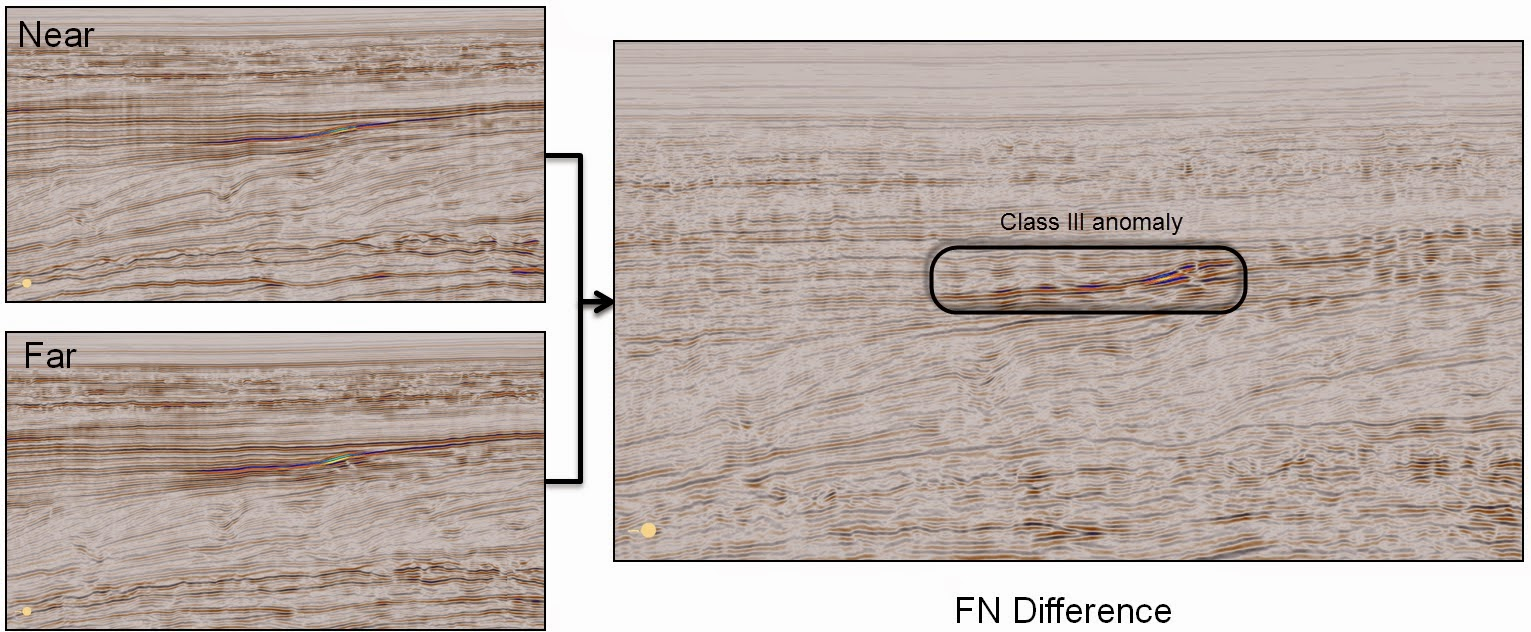Luis Gomez | April 23, 2015

## AVO Screening (Part 1): Difference and ratio volumesLuis Gomez

Related Topics:

The simplest way of AVO Screening involves the computation of difference and ratio volumes to highlight differences in amplitude between stacks. This amplitude difference may represent lithological/fluid differences.

Class III anomalies are usually highlighted by applying a simple Far-Near difference volume (Ross & Kinman, 1995) or sometimes a (Far-Near)*Far.Far-Near difference volume calculated on the Rosewall-Calliance dataset (NW shelf of Australia)

When loading the angle stacks into Geoteric, we advise to use User scale and carefully look at the amplitude range of the input volumes so that the amplitude relationships are preserved.

The difference volumes can be calculated for any attribute or even directly on the reflectivity. We have found the envelope difference volume to be quite effective, as that way phase is ignored and we can see contiguous regions of difference.

In Geoteric, we use the Parser to calculate these volumes. The Parser can be found in Processes & Workflows > Processes > Volume Maths > Parser.

To calculate a simple difference volume we can just select our two input volumes and type the expression im1-im2.

The ratio volume can be calculated using ((im1/im2)-1* scale). The scale factor will need to be adjusted depending on the data type and dynamic range of the input volumes.

The other parts can be found here: part 2 , part 3 , part 4, part 5